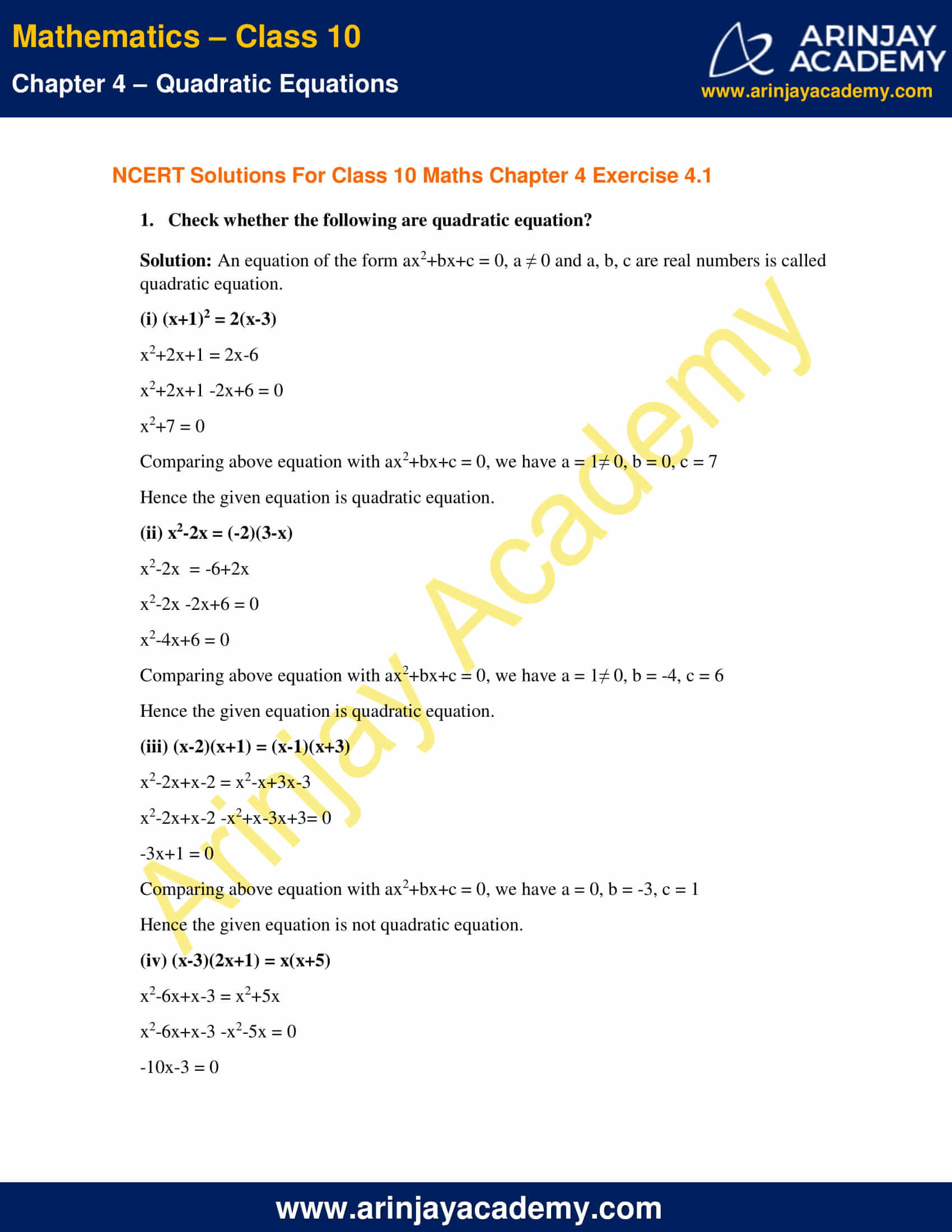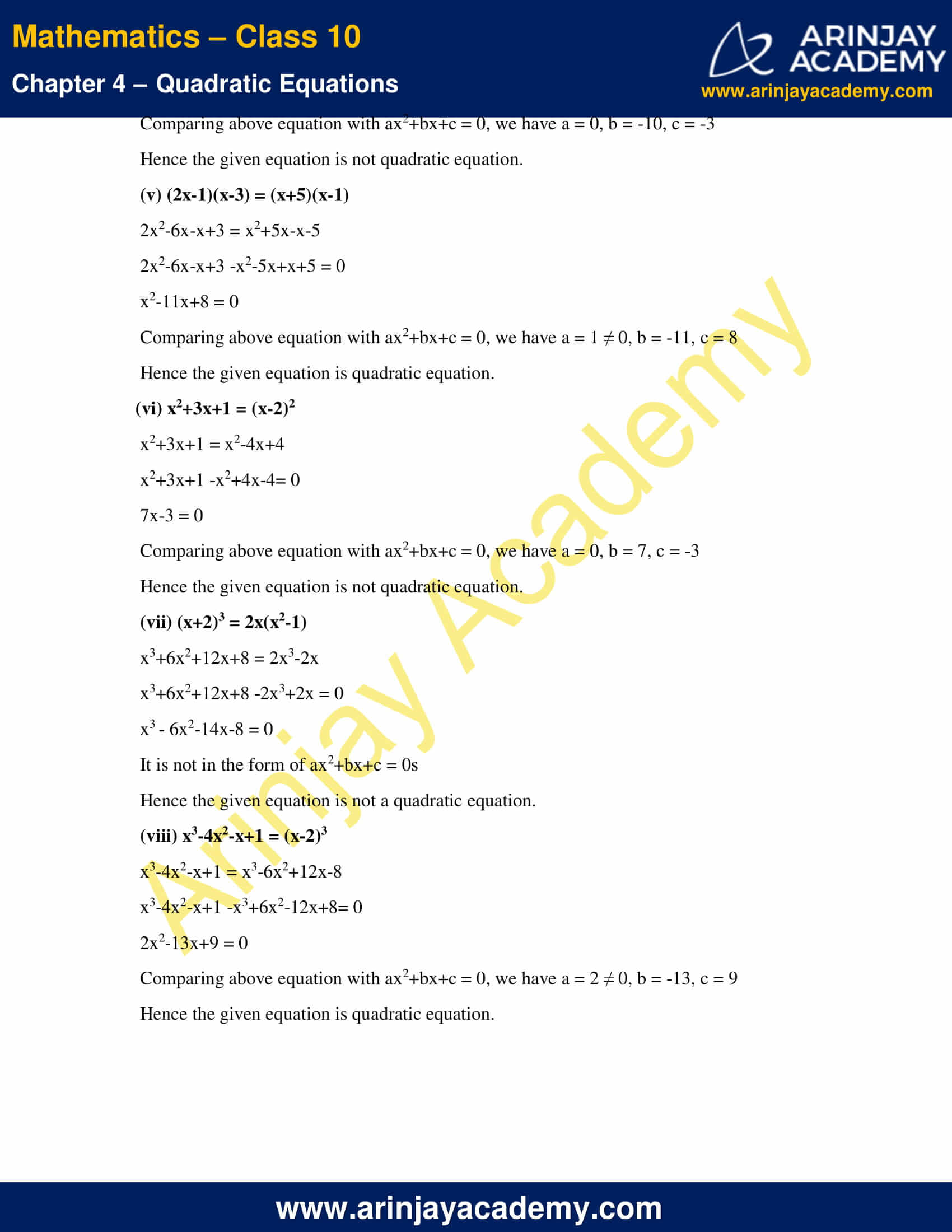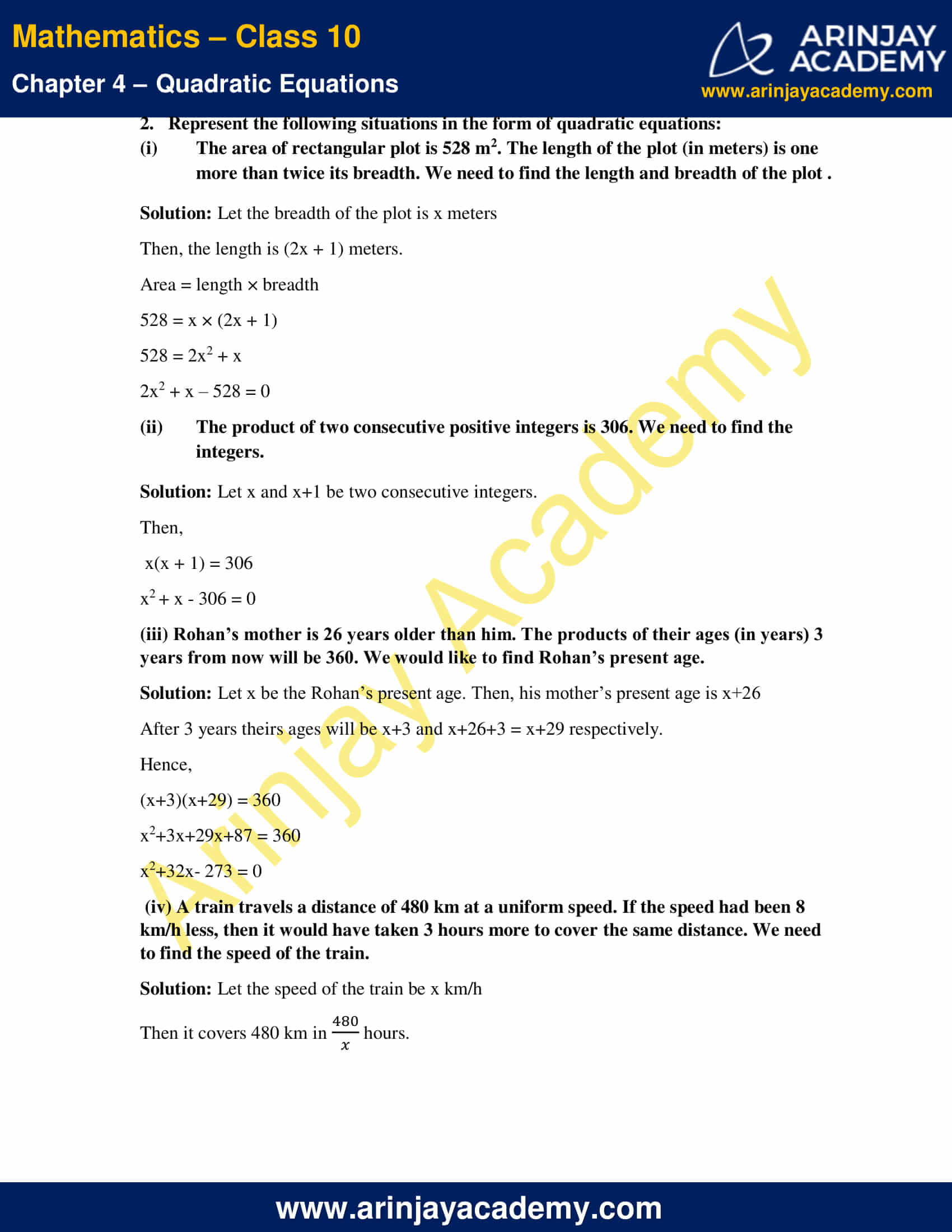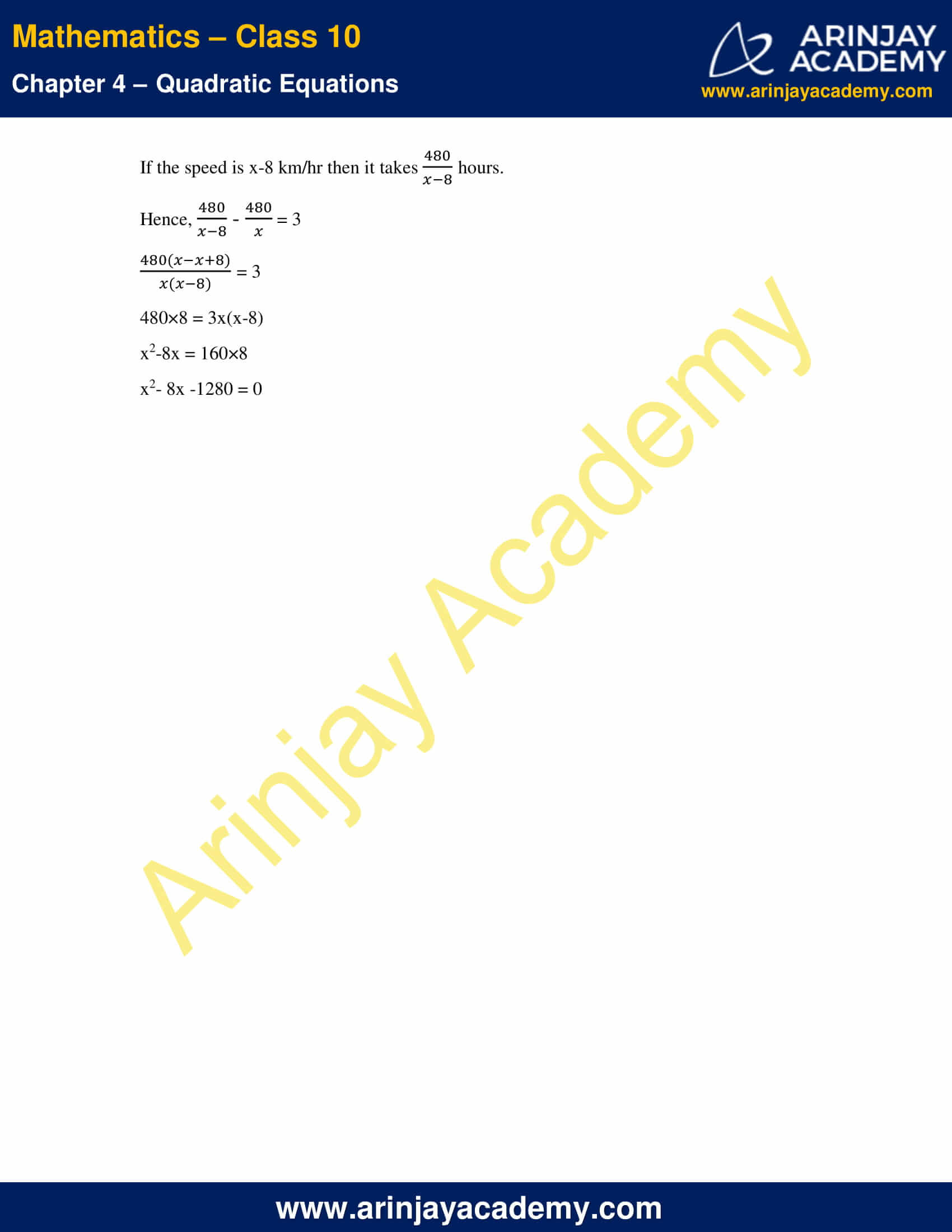# NCERT Solutions For Class 10 Maths Chapter 4 Exercise 4.1 – Quadratic Equations

Download NCERT Solutions For Class 10 Maths Chapter 4 Exercise 4.1 – Quadratic Equations. This Exercise contains 2 questions, for which detailed answers have been provided in this note. In case you are looking at studying the remaining Exercise for Class 10 for Maths NCERT solutions for other Chapters, you can click the link at the end of this Note.

### NCERT Solutions For Class 10 Maths Chapter 4 Exercise 4.1 – Quadratic EquationsNCERT Solutions For Class 10 Maths Chapter 4 Exercise 4.1 – Quadratic Equations

1. Check whether the following are quadratic equation?

Solution: An equation of the form ax2+bx+c = 0, a ≠ 0 and a, b, c are real numbers is called quadratic equation.

(i) (x+1)2 = 2(x-3)

x2+2x+1 = 2x-6
x2+2x+1 -2x+6 = 0
x2+7 = 0
Comparing above equation with ax2+bx+c = 0, we have a = 1≠ 0, b = 0, c = 7
Hence the given equation is quadratic equation.

(ii) x2-2x = (-2)(3-x)

x2-2x  = -6+2x
x2-2x -2x+6 = 0
x2-4x+6 = 0
Comparing above equation with ax2+bx+c = 0, we have a = 1≠ 0, b = -4, c = 6
Hence the given equation is quadratic equation.

(iii) (x-2)(x+1) = (x-1)(x+3)

x2-2x+x-2 = x2-x+3x-3
x2-2x+x-2 -x2+x-3x+3= 0
-3x+1 = 0
Comparing above equation with ax2+bx+c = 0, we have a = 0, b = -3, c = 1
Hence the given equation is not quadratic equation.

(iv) (x-3)(2x+1) = x(x+5)

x2-6x+x-3 = x2+5x
x2-6x+x-3 -x2-5x = 0
-10x-3 = 0
Comparing above equation with ax2+bx+c = 0, we have a = 0, b = -10, c = -3
Hence the given equation is not quadratic equation.

(v) (2x-1)(x-3) = (x+5)(x-1)

2x2-6x-x+3 = x2+5x-x-5
2x2-6x-x+3 -x2-5x+x+5 = 0
x2-11x+8 = 0
Comparing above equation with ax2+bx+c = 0, we have a = 1 ≠ 0, b = -11, c = 8
Hence the given equation is quadratic equation.

(vi) x2+3x+1 = (x-2)2

x2+3x+1 = x2-4x+4
x2+3x+1 -x2+4x-4= 0
7x-3 = 0
Comparing above equation with ax2+bx+c = 0, we have a = 0, b = 7, c = -3
Hence the given equation is not quadratic equation.

(vii) (x+2)3 = 2x(x2-1)

x3+6x2+12x+8 = 2x3-2x
x3+6x2+12x+8 -2x3+2x = 0
x3 – 6x2-14x-8 = 0
It is not in the form of ax2+bx+c = 0s
Hence the given equation is not a quadratic equation.

(viii) x3-4x2-x+1 = (x-2)3

x3-4x2-x+1 = x3-6x2+12x-8
x3-4x2-x+1 -x3+6x2-12x+8= 0
2x2-13x+9 = 0
Comparing above equation with ax2+bx+c = 0, we have a = 2 ≠ 0, b = -13, c = 9
Hence the given equation is quadratic equation.

2. Represent the following situations in the form of quadratic equations:

(i) The area of rectangular plot is 528 m2. The length of the plot (in meters) is one more than twice its breadth. We need to find the length and breadth of the plot .

Solution: Let the breadth of the plot is x meters
Then, the length is (2x + 1) meters.
528 = x × (2x + 1)
528 = 2x2 + x
2x2 + x – 528 = 0

(b) The product of two consecutive positive integers is 306. We need to find the integers.

Solution: Let x and x+1 be two consecutive integers.
Then, x(x + 1) = 306
x2 + x – 306 = 0

(iii) Rohan’s mother is 26 years older than him. The products of their ages (in years) 3 years from now will be 360. We would like to find Rohan’s present age.

Solution: Let x be the Rohan’s present age. Then, his mother’s present age is x+26
After 3 years theirs ages will be x+3 and x+26+3 = x+29 respectively.
Hence,
(x+3)(x+29) = 360
x2+3x+29x+87 = 360
x2+32x- 273 = 0

(iv) A train travels a distance of 480 km at a uniform speed. If the speed had been 8 km/h less, then it would have taken 3 hours more to cover the same distance. We need to find the speed of the train.

Solution: Let the speed of the train be x km/h
Then it covers 480 km in (480/x) hours.
If the speed is x-8 km/hr then it takes (480/x-8) hours.
Hence, (480/x-8) – (480/x) = 3
[480(x-x+8)/x(x-8)] = 3
480×8 = 3x(x-8)
x2-8x = 160×8
x2– 8x -1280 = 0

NCERT Solutions for Class 10 Maths Chapter 4 Exercise 4.1 – Quadratic Equations, has been designed by the NCERT to test the knowledge of the student on the topic – Quadratic Equations